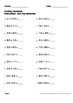## Adding and Subtracting Decimals Worksheets\$4.00Buy This Resource Now

Adding and Subtracting Decimals package includes 200 worksheets.

Addition. 1 decimal place + 1 decimal place (20 worksheets)
Subtraction. 1 decimal place - 1 decimal place (20 worksheets)
Addition. 2 decimal places + 1 decimal place (20 worksheets)
Subtraction. 2 decimal places - 1 decimal place (20 worksheets)
Addition. 2 decimal places + 2 decimal places (20 worksheets)
Subtraction. 2 decimal places - 2 decimal places (20 worksheets)
Vertical Addition. 2 decimal place + 1 decimal place (20 worksheets)
Vertical Subtraction. 2 decimal places - 1 decimal place (20 worksheets)
Vertical Addition. 2 decimal places + 2 decimal places (20 worksheets)
Vertical Subtraction. 2 decimal places - 2 decimal places (20 worksheets)

You can purchase Decimals Worksheets Bundle and save your money!
Decimals Worksheets Bundle

----------------------------------

You May Also Like These Worksheets:
Adding and Subtracting Decimals
Multiplying Decimals Worksheets
Dividing Decimals Worksheets
Decimals and Percents Worksheets
Decimals on a Numberlines
Identify Decimals Worksheets
Ordering Decimals Worksheets
Comparing Decimals Worksheets
Decimals to Word Form
Decimals [Bundle!]

You May Also Like These Task Cards:
Comparing Decimals Task Cards
Subtracting Decimals Task Cards
Multiplying Decimals Task Cards
Dividing Decimals Task Cards
Decimals to Word Form Task Cards
Ordering Decimals Task Cards
Decimals and Percents Task Cards
Identify Decimals Task Cards
Decimals on a Number Line Task Card
Decimals Task Cards Bundle (Bundle)

Be the first to know about my new discounts, freebies and product launches:
- Look for the green star next to my store logo and click it to become a follower. You will now receive email updates about this store.